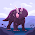## Rein's rate problem

http://www.livestrong.com/article/490366-how-to-eliminate-gas-and-bloating-with-apples-and-milk/

Group Problem
rate to unit rate
If there's 6 girls per 2 boys, how many girl if there's only 1 boy?

My Problem
rate to unit rate
There are \$0.25 per 100 ml of milk, how many cents there are in ml of milk?

My problem
unit rate to rate
If there's 20 apples per milk, how many apples there are in 50 pieces of milk?

Group Problem
unit rate to rate
If there's 2 paper per pencil, how many paper are there if there's 10 pencils?

1:58 PM

#### 4 comments :

1.4). the answer is 20 papers per 10 pencils
changing questions: If there's 3 paper per pencil, how many paper are there if there's 30 pencils?

2.1). If there's only 1 boy, there would be 3 girls, because 6/2 is 3 which are the number of girls if there's only 1 boy.

Your Word Problem: If there's 20 girls and 10 boys, how many boys are there if there are 10 girls?

3.the answer for Rosheena's question is 60 paper per 30 pencils because 20/2=10 and 60/2=30 X=60

4.the answer for Ileana's question is 5 boys per 10 girls because 20/2=10 and then 10/2=5 X=5 boys